• 本系统是信息检索课程的一个关于文本相似度对比的程序，它是用Java设计的，你也可以直接点击里面的jar文件进行运行，本程序还可以用来防止同学进行文档作弊的作业，资源里面有还有它的使用方法。
• 使用java调用HanLP分词器实现两个文本相似度对比，可以很快对比出百分率（1=100%）
• 易语言文本相似度比较，逐字比较，是把第一个字符串每个字都拆分开来和第二个字符串相比较
• ## 文本相似度比较

热门讨论 2012-08-27 12:19:46
能够对text文档进行语义相似度比较，并能进行术语之间的相似度计算。
• 使用java实现输出文本相似度的一些代码。能够运行，有结果有数据。
• 文本相似度比较，java实现，文本相似度比较，java实现java实现java实现
• ## 文本相似度对比

千次阅读 2019-01-16 18:28:15
import numpy as np from scipy.linalg import norm from scipy import stats from sklearn.feature_extraction.text import CountVectorizer, TfidfVectorizer def jaccard_similarity(s1, s2): ...
import numpy as np
from scipy.linalg import norm
from scipy import stats
from sklearn.feature_extraction.text import CountVectorizer, TfidfVectorizer
def jaccard_similarity(s1, s2):
vectorizer = CountVectorizer(tokenizer=lambda s: s.split())
corpus = [s1, s2]
vectors = vectorizer.fit_transform(corpus).toarray()
numerator = np.sum(np.min(vectors, axis=0))
denominator = np.sum(np.max(vectors, axis=0))
return 1.0 * numerator / denominator

def cosine_similarity_tf(s1, s2):

vectorizer = CountVectorizer(tokenizer=lambda s: s.split())
corpus = [s1, s2]
vectors = vectorizer.fit_transform(corpus).toarray()
return np.dot(vectors, vectors) / (norm(vectors) * norm(vectors))

def cosine_similarity_tfidf(s1, s2):

vectorizer = TfidfVectorizer(tokenizer=lambda s: s.split())
corpus = [s1, s2]
vectors = vectorizer.fit_transform(corpus).toarray()
# print(vectors,vectors)
return np.dot(vectors, vectors) / (norm(vectors) * norm(vectors))

params = ['新 疆 生 产 建 设 兵 团 阿 克 苏 垦 区 人 民 检 察 院', '新 疆 产 建 设 兵 团 阿 克 苏 垦 区 ']
print(jaccard_similarity(*params))
print(cosine_similarity_tf(*params))
print(cosine_similarity_tfidf(*params))


展开全文• 杰卡德相似系数是衡量两个集合相似度的一种指标（余弦距离也可以用来衡量两个集合的相似度）。 clip_image013 （2）杰卡德距离 与杰卡德相似系数相反的概念是杰卡德距离（Jaccard Distance），可以用如下公...
杰卡德相似性度量
（1）杰卡德相似系数
两个集合A和B交集元素的个数在A、B并集中所占的比例，称为这两个集合的杰卡德系数，用符号 J(A,B) 表示。杰卡德相似系数是衡量两个集合相似度的一种指标（余弦距离也可以用来衡量两个集合的相似度）。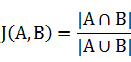clip_image013

（2）杰卡德距离
与杰卡德相似系数相反的概念是杰卡德距离（Jaccard Distance），可以用如下公式来表示：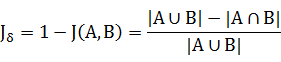clip_image015

杰卡德距离用两个两个集合中不同元素占所有元素的比例来衡量两个集合的区分度。
（3）杰卡德相似系数的应用
假设样本A和样本B是两个n维向量，而且所有维度的取值都是0或1。例如，A（0,1,1,0）和B（1,0,1,1）。我们将样本看成一个集合，1表示集合包含该元素，0表示集合不包含该元素。
p：样本A与B都是1的维度的个数
q：样本A是1而B是0的维度的个数
r：样本A是0而B是1的维度的个数
s：样本A与B都是0的维度的个数
那么样本A与B的杰卡德相似系数可以表示为：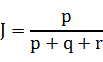clip_image017

此处分母之所以不加s的原因在于：
对于杰卡德相似系数或杰卡德距离来说，它处理的都是非对称二元变量。非对称的意思是指状态的两个输出不是同等重要的，例如，疾病检查的阳性和阴性结果。
按照惯例，我们将比较重要的输出结果，通常也是出现几率较小的结果编码为1（例如HIV阳性），而将另一种结果编码为0（例如HIV阴性）。给定两个非对称二元变量，两个都取1的情况（正匹配）认为比两个都取0的情况（负匹配）更有意义。负匹配的数量s认为是不重要的，因此在计算时忽略。
（4）杰卡德相似度算法分析
杰卡德相似度算法没有考虑向量中潜在数值的大小，而是简单的处理为0和1，不过，做了这样的处理之后，杰卡德方法的计算效率肯定是比较高的，毕竟只需要做集合操作。
Needleman-Wunsch
基于最长公共子串的文本比较算法——Needleman/Wunsch算法,用的都是动态规划的思想。在Needleman/Wunsch算法中还设定了一个权值，用以区分三种操作（插入、删除、更改）的优先级。
关键点1. 打分矩阵： 选用不同的打分矩阵或者罚分分值会导致比对结果不同，常用BLAST打分矩阵。2. 计算比对最高得分的算法： 常用动态规划算法（Needleman-Wunsch算法）。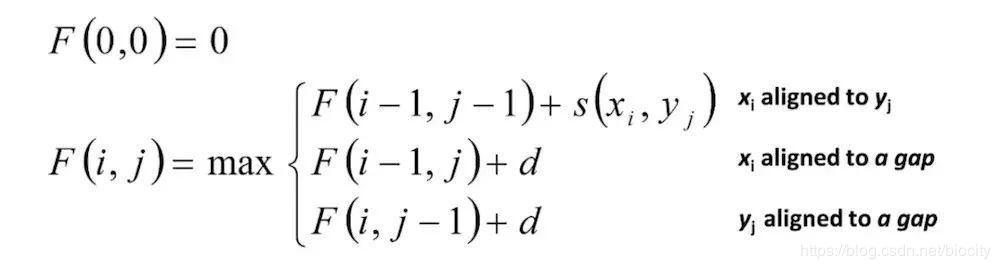在这里插入图片描述

打印出最高得分相应的序列比对结果： 根据得分矩阵回溯，如果最优比对结果有多个，全部打印出来。理解打分系统背后的概率论模型： 比对分值可以理解为匹配模型和随机模型的对数几率比（log-odds ratio）。
Jaro–Winkler similarity
levenshtein距离是编辑距离的其中一种定义，本文所说的Jaro距离是编辑距离的另外一种定义，它也是对两个字符串的相似度进行衡量，以得出两字符串的相似程度。 1、Jaro distance/similarity 对于两个字符串s1和s2，它们的Jaro 相似度由下面公式给出：
其中： ①|s1|和|s2|表示字符串s1和s2的长度。 ②m表示两字符串的匹配字符数。 ③t表示换位数目transpositions的一半。
这里的m和t是满足一定条件下得出来的，在理解m和t的含义之前，我们先来认识匹配窗口(记为matching window，mw)的概念。Jaro算法的字符之间的比较是限定在一个范围内的，如果在这个范围内两个字符相等，那么表示匹配成功，如果超出了这个范围，表示匹配失败。而这个范围就是匹配窗口，在Jaro算法中，它被定义为不超过下面表达式的值：
比如说字符串A(“bacde”)和B(“abed”)，它的匹配窗口大小为1，在匹配的过程中，字符’a’、‘b’、‘d’都是匹配的，indexInA(‘d’) = 3，indexInB(‘d’) = 3，二者的距离是0，小于匹配窗口大小。但对于’e’，虽然两字符串都有’e’这个字符，但它们却是不匹配的，因为’e’的下标分别为4和2，距离为2 > mw，所以’e’是不匹配的。在这个例子中，由于有3个字符匹配，因此m = 3。换位数目表示不同顺序的匹配字符的个数。同样看这个例子，'a’和’b’都是匹配的，但’a’和’b’在两个字符串的表示为"ba…“和"ab…”，它们的顺序不同，因此这里换位数目transpositions = 2，而t = transpositions / 2 = 1。
对于匹配窗口的含义，笔者的理解是：匹配窗口是一个阈值，在这个阈值之内两个字符相等，可以认为是匹配的；超过了这个阈值，即使存在另一个字符与该字符相等，但由于它们的距离太远了，二者的相关性太低了，不能认为它们是匹配的。从上面的公式可以看出，该算法强调的是局部相似度。
对于任意字符串A和B，能求出它们的length、m和t，这样便能代入公式求得二者的相似度(Jaro similarity)。从刚才的例子得到，|s1|=5，|s2|=4，m=3，t=1，代入公式可得：simj = (3/5 + 3/4 + (3-1)/3)/3 = 0.672
2、Jaro-Winkler distance/similarity Jaro-Winkler similarity是在Jaro similarity的基础上，做的进一步修改，在该算法中，更加突出了前缀相同的重要性，即如果两个字符串在前几个字符都相同的情况下，它们会获得更高的相似性。该算法的公式如下：
其中： ①simj 就是刚才求得的Jaro similarity。 ②l表示两个字符串的共同前缀字符的个数，最大不超过4个。 ③p是缩放因子常量，它描述的是共同前缀对于相似度的贡献，p越大，表示共同前缀权重越大，最大不超过0.25。p默认取值是0.1。
图解Jaro-Winkler similarity求解过程 下面以字符串A(“abcdefgh”)和字符串B(“abehc”)为例来介绍整个算法的流程。这里以短字符串为行元素，长字符串为列元素，建立(|s1|+1)×(|s2|+1)的矩阵，这里匹配窗口的大小为3(注意包括距离为0的匹配)，然后根据公式不断运算：
从上面的图以及公式，我们可以总结出求解的过程：字符串s1作为行元素，字符串s2作为列元素，窗口大小为mw，同时建立两个布尔型数组，大小分别为s1和s2的长度，布尔型数组对应下标的值True表示已匹配，false表示不匹配。 对于行元素的每一个字符c1，根据c1在该字符串s1中的下标k，定位到s2的k位置，然后在该位置往前遍历mw个单位，往后遍历mw个单位，如果寻找到相等的字符，记在s2中的下标为p。经过这样的一次遍历，找到了k和p，我们分别标记布尔型数组s1的k和布尔型数组s2的p为已匹配(true)，下次遍历时就跳过该已匹配的字符。当对s1的所有元素都遍历完毕时，就找到了所有已匹配的字符，我们统计已匹配的字符便能得到m，然后对两个布尔型数组同时按照顺序比较，如果出现了true，但二者对应字符串相应位置的字符不相等，表示这是非顺序的匹配，这样就可以得到t。这样就能根据m和t求出Jaro similarity了。至于Jaro-Winkler similarity，需要p参数，也不难，求出俩字符串最大共同前缀的大小即可。 如果读者对上面的过程还有疑问，笔者再提一点，关键就在于判断来自俩字符串的相等字符的距离是不是超过了阈值(即匹配窗口长度)。这里的判断方法是在某个位置进行前后的搜索，包括当前位置。
展开全文机器学习
• 中文文本相似度匹配算法 simHash 海明距离 IK分词 完整的可运行的示例代码 包含simHash 算法，使用IK 对中文文本进行分词处理
• ## 文本相似度算法的对比及python实现

千次阅读 多人点赞 2019-11-01 15:00:42
文本相似度算法的对比及python实现 前言 通常我们有这样的需求：对两篇文章或者产品内容进行重复率查询。 为了解决类似的问题，罗列了一些常见的相似度算法，用python代码实现。 五种常见的相似度算法：余弦相似度...
文本相似度算法的对比及python实现
前言
通常我们有这样的需求：对两篇文章或者产品内容进行重复率查询。
为了解决类似的问题，罗列了一些常见的相似度算法，用python代码实现。
五种常见的相似度算法：余弦相似度（cosine_similarity）、jaccard相似度、编辑距离（Levenshtein）、MinHash、SimHash + 海明距离。
代码是一位前辈留下的，做一下整理分享出来。算法的具体理论这里就不硬搬生套了，大家可以自行搜索。有任何问题欢迎留言，谢谢！
余弦相似度cosine_similarity
# -*- coding: utf-8 -*-

# 正则包
import re
# html 包
import html
# 自然语言处理包
import jieba
import jieba.analyse
# 机器学习包
from sklearn.metrics.pairwise import cosine_similarity

class CosineSimilarity(object):
"""
余弦相似度
"""
def __init__(self, content_x1, content_y2):
self.s1 = content_x1
self.s2 = content_y2

@staticmethod
def extract_keyword(content):  # 提取关键词
# 正则过滤 html 标签
re_exp = re.compile(r'(<style>.*?</style>)|(<[^>]+>)', re.S)
content = re_exp.sub(' ', content)
# html 转义符实体化
content = html.unescape(content)
# 切割
seg = [i for i in jieba.cut(content, cut_all=True) if i != '']
# 提取关键词
keywords = jieba.analyse.extract_tags("|".join(seg), topK=200, withWeight=False)
return keywords

@staticmethod
def one_hot(word_dict, keywords):  # oneHot编码
# cut_code = [word_dict[word] for word in keywords]
cut_code = *len(word_dict)
for word in keywords:
cut_code[word_dict[word]] += 1
return cut_code

def main(self):
# 去除停用词
jieba.analyse.set_stop_words('./files/stopwords.txt')

# 提取关键词
keywords1 = self.extract_keyword(self.s1)
keywords2 = self.extract_keyword(self.s2)
# 词的并集
union = set(keywords1).union(set(keywords2))
# 编码
word_dict = {}
i = 0
for word in union:
word_dict[word] = i
i += 1
# oneHot编码
s1_cut_code = self.one_hot(word_dict, keywords1)
s2_cut_code = self.one_hot(word_dict, keywords2)
# 余弦相似度计算
sample = [s1_cut_code, s2_cut_code]
# 除零处理
try:
sim = cosine_similarity(sample)
return sim
except Exception as e:
print(e)
return 0.0

# 测试
if __name__ == '__main__':
with open('./files/sample_x.txt', 'r') as x, open('./files/sample_y.txt', 'r') as y:
similarity = CosineSimilarity(content_x, content_y)
similarity = similarity.main()
print('相似度: %.2f%%' % (similarity*100))


输出结果：
Building prefix dict from the default dictionary ...
Prefix dict has been built succesfully.
相似度: 79.67%

jaccard相似度
# -*- coding: utf-8 -*-

# 正则包
import re
# 自然语言处理包
import jieba
import jieba.analyse
# html 包
import html

class JaccardSimilarity(object):
"""
jaccard相似度
"""
def __init__(self, content_x1, content_y2):
self.s1 = content_x1
self.s2 = content_y2

@staticmethod
def extract_keyword(content):  # 提取关键词
# 正则过滤 html 标签
re_exp = re.compile(r'(<style>.*?</style>)|(<[^>]+>)', re.S)
content = re_exp.sub(' ', content)
# html 转义符实体化
content = html.unescape(content)
# 切割
seg = [i for i in jieba.cut(content, cut_all=True) if i != '']
# 提取关键词
keywords = jieba.analyse.extract_tags("|".join(seg), topK=200, withWeight=False)
return keywords

def main(self):
# 去除停用词
jieba.analyse.set_stop_words('./files/stopwords.txt')

# 分词与关键词提取
keywords_x = self.extract_keyword(self.s1)
keywords_y = self.extract_keyword(self.s2)

# jaccard相似度计算
intersection = len(list(set(keywords_x).intersection(set(keywords_y))))
union = len(list(set(keywords_x).union(set(keywords_y))))
# 除零处理
sim = float(intersection)/union if union != 0 else 0
return sim

# 测试
if __name__ == '__main__':
with open('./files/sample_x.txt', 'r') as x, open('./files/sample_y.txt', 'r') as y:
similarity = JaccardSimilarity(content_x, content_y)
similarity = similarity.main()
print('相似度: %.2f%%' % (similarity*100))


输出结果：
Building prefix dict from the default dictionary ...
Prefix dict has been built succesfully.
相似度: 66.20%

编辑距离Levenshtein
# -*- coding: utf-8 -*-

# 正则包
import re
# html 包
import html
# 自然语言处理包
import jieba
import jieba.analyse
# 编辑距离包
import Levenshtein

class LevenshteinSimilarity(object):
"""
编辑距离
"""
def __init__(self, content_x1, content_y2):
self.s1 = content_x1
self.s2 = content_y2

@staticmethod
def extract_keyword(content):  # 提取关键词
# 正则过滤 html 标签
re_exp = re.compile(r'(<style>.*?</style>)|(<[^>]+>)', re.S)
content = re_exp.sub(' ', content)
# html 转义符实体化
content = html.unescape(content)
# 切割
seg = [i for i in jieba.cut(content, cut_all=True) if i != '']
# 提取关键词
keywords = jieba.analyse.extract_tags("|".join(seg), topK=200, withWeight=False)
return keywords

def main(self):
# 去除停用词
jieba.analyse.set_stop_words('./files/stopwords.txt')

# 提取关键词
keywords1 = ', '.join(self.extract_keyword(self.s1))
keywords2 = ', '.join(self.extract_keyword(self.s2))

# ratio计算2个字符串的相似度，它是基于最小编辑距离
distances = Levenshtein.ratio(keywords1, keywords2)
return distances

# 测试
if __name__ == '__main__':
with open('./files/sample_x.txt', 'r') as x, open('./files/sample_y.txt', 'r') as y:
distance = LevenshteinSimilarity(content_x, content_y)
distance = distance.main()
print('相似度: %.2f%%' % (distance * 100))


输出结果：
Building prefix dict from the default dictionary ...
Prefix dict has been built succesfully.
相似度: 82.24%

MinHash
# -*- coding: utf-8 -*-

# 正则包
import re
# 自然语言处理包
import jieba
import jieba.analyse
# html 包
import html
# 数据集处理包

class MinHashSimilarity(object):
"""
MinHash
"""
def __init__(self, content_x1, content_y2):
self.s1 = content_x1
self.s2 = content_y2

@staticmethod
def extract_keyword(content):  # 提取关键词
# 正则过滤 html 标签
re_exp = re.compile(r'(<style>.*?</style>)|(<[^>]+>)', re.S)
content = re_exp.sub(' ', content)
# html 转义符实体化
content = html.unescape(content)
# 切割
seg = [i for i in jieba.cut(content, cut_all=True) if i != '']
# 提取关键词
keywords = jieba.analyse.extract_tags("|".join(seg), topK=200, withWeight=False)
return keywords

def main(self):
# 去除停用词
jieba.analyse.set_stop_words('./files/stopwords.txt')

# MinHash计算
m1, m2 = MinHash(), MinHash()
# 提取关键词
s1 = self.extract_keyword(self.s1)
s2 = self.extract_keyword(self.s2)

for data in s1:
m1.update(data.encode('utf8'))
for data in s2:
m2.update(data.encode('utf8'))

return m1.jaccard(m2)

# 测试
if __name__ == '__main__':
with open('./files/sample_x.txt', 'r') as x, open('./files/sample_y.txt', 'r') as y:
similarity = MinHashSimilarity(content_x, content_y)
similarity = similarity.main()
print('相似度: %.2f%%' % (similarity*100))


输出结果：
Building prefix dict from the default dictionary ...
Prefix dict has been built succesfully.
相似度: 64.84%

SimHash + 海明距离
# -*- coding: utf-8 -*-

# 正则
import re
# html 包
import html
# 数学包
import math
# 自然语言处理包
import jieba
import jieba.analyse

class SimHashSimilarity(object):
"""
SimHash
"""
def __init__(self, content_x1, content_y2):
self.s1 = content_x1
self.s2 = content_y2

@staticmethod
def get_bin_str(source):  # 字符串转二进制
if source == "":
return 0
else:
t = ord(source) << 7
m = 1000003
mask = 2 ** 128 - 1
for c in source:
t = ((t * m) ^ ord(c)) & mask
t ^= len(source)
if t == -1:
t = -2
t = bin(t).replace('0b', '').zfill(64)[-64:]
return str(t)

@staticmethod
def extract_keyword(content):  # 提取关键词
# 正则过滤 html 标签
re_exp = re.compile(r'(<style>.*?</style>)|(<[^>]+>)', re.S)
content = re_exp.sub(' ', content)
# html 转义符实体化
content = html.unescape(content)
# 切割
seg = [i for i in jieba.cut(content, cut_all=True) if i != '']
# 提取关键词
keywords = jieba.analyse.extract_tags("|".join(seg), topK=200, withWeight=True)
return keywords

def run(self, keywords):
ret = []
for keyword, weight in keywords:
bin_str = self.get_bin_str(keyword)
key_list = []
for c in bin_str:
weight = math.ceil(weight)
if c == "1":
key_list.append(int(weight))
else:
key_list.append(-int(weight))
ret.append(key_list)
# 对列表进行"降维"
rows = len(ret)
cols = len(ret)
result = []
for i in range(cols):
tmp = 0
for j in range(rows):
tmp += int(ret[j][i])
if tmp > 0:
tmp = "1"
elif tmp <= 0:
tmp = "0"
result.append(tmp)
return "".join(result)

def main(self):
# 去除停用词
jieba.analyse.set_stop_words('./files/stopwords.txt')

# 提取关键词
s1 = self.extract_keyword(self.s1)
s2 = self.extract_keyword(self.s2)

sim_hash1 = self.run(s1)
sim_hash2 = self.run(s2)
# print(f'相似哈希指纹1: {sim_hash1}\n相似哈希指纹2: {sim_hash2}')
length = 0
for index, char in enumerate(sim_hash1):
if char == sim_hash2[index]:
continue
else:
length += 1
return length

# 测试
if __name__ == '__main__':
with open('./files/sample_x.txt', 'r') as x, open('./files/sample_y.txt', 'r') as y:

similarity = SimHashSimilarity(content_x, content_y)
similarity = similarity.main()
# 阀值
threshold = 3
print(f'海明距离：{similarity} 判定距离：{threshold} 是否相似：{similarity <= threshold}')


输出结果：
Building prefix dict from the default dictionary ...
Prefix dict has been built succesfully.
海明距离：17 判定距离：3 是否相似：False

讨论
几种算法对比：
余弦相似度 计算复杂度偏高。 相关研究中，基于物品协同过滤系统的相似性度量方法普遍使用余弦相似性。 然而，在许多实际应用中,数据稀疏度过高，通过余弦相似度计算会产生误导性结果。  jaccard相似度 在产品描述中，很多运营人员为了偷懒，喜欢复制粘贴稍作修改，造成产品描述重复度高。通过提取产品描述的关键词，再计算两组关键词的交集并集非常适合在此场景下检测产品描述的重复度，即杰卡德相似度。  编辑距离 计算复杂度偏高，且在此场景效果并不理想。  MinHash 在大数据集中求杰尔德相似度的解决方案，通过对数据文本的降维，大大提高计算速度。  SimHash + 海明距离 对单词数量低于500的文章误差较大。
MinHash与SimHash的区别：https://blog.csdn.net/dm_ustc/article/details/45626907
根据需求场景，选择合适的算法，或者集成算法。代码详情见github：https://github.com/downdawn/Similarity
笔记，有任何问题欢迎留言，谢谢！
展开全文• 创建文档摘要向量之后，可通过比较两个文档摘要向量的距离的方法来判断两个文档的相似度
推荐自己的专栏：分享一些Python案例，将所学用出来

一：文本相似度比较概述
通过计算并比较文档的摘要，可实现文本的相似度比较。
文档摘要的最简单形式可以使用文档中的k-grams（k个连续字符）的相对频率的向量来表示。假设字符的取值可能有128种不同的值(ASCII码) ，则向量的维度d为128k；对于Unicode编码，这更是天文数字。因此，一般使用哈希函数hash(s) % d把k-grams字符串s映射到0到d-1之间的整数，从而使得文档摘要向量的维度为d。.创建文档摘要向量之后，可通过比较两个文档摘要向量的距离的方法来判断两个文档的相似度。
下面先阐述向量类（Vector） 和文档摘要类（Sketch）的设计与实现，然后使用文档摘要类来比较文档的相似度。
二：向量(Vector) 类设计和实现
向量是一种数学抽象，n维向量可以使用一个n个实数的有序列表： (x0, x1,…,xn-1)。向量支持基本的四则算数运算，故可通过运算符重载来实现。
向量的基本运算 包括：两个向量的加法、一个向量乘以一个标量（一个实数）、计算两个向量的点积、计算向量大小和方向。
加法：x+y = (x0+y0, x1+y1, …, xn-1+yn-1)减法：x-y = (x0-y0，x1-y1，…，xn-1-yn-1)标量积：ax = (ax0，ax1, …，axn-1)点积：xy = x0y0 + x1y1 +… + xn-1yn-1大小：|x| = (x02 + x12 + … + xn-12)1/2方向：x / |x| = ( x0 / |x|，x1 / |x|，…，xn-1 / |x| )
基本的向量(Vector) 类设计思路如下：
定义带一个列表参数(向量的坐标，可以是任意维度)的构造函数，用于初始化对应向量的实例对象属性：_coords。
def __init__(self, a):
"""构造函数：切片拷贝列表参数a到对象实例变量_coords"""
self._coords = a[:]
self._n = len(a)

重载方法__getitem__ ，返回第 i 个元素，即第 i 维坐标。
def __getitem__(self, i):
"""返回第i个元素，即第i维坐标"""
return self._coords[i]

重载方法__len__， 返回向量的维度。
def __len__(self):
"""返回向量的维度"""
return self._n

重载方法__str__ ，返回向量的字符串表示。.
def __str__(self):
"""返回向量的字符串表示"""
return str(self._coords)

重载方法__add__ 、__sub__ ，实现向量的运算：加法、减法。
加法：x+y = (x0+y0, x1+y1, …, xn-1+yn-1) 减法：x-y = (x0-y0，x1-y1，…，xn-1-yn-1)
def __add__(self, other):
"""返回2个向量之和"""
result = []
for i in range(self._n):
result.append(self._coords[i] + other._coords[i])
return Vector(result)

def __sub__(self, other):
"""返回2个向量之差"""
result = []
for i in range(self._n):
result.append(self._coords[i] - other._coords[i])
return Vector(result)

定义方法scale()，实现向量的运算：标量积。
标量积：ax = (ax0，ax1, …，axn-1)
def scale(self, n):
"""返回向量与数值的乘积（标量积）"""
result = []
for i in range(self._n):
result.append(self._coords[i] * n)
return Vector(result)

定义方法 dot()，实现向量的运算：点积。
点积：xy = x0y0 + x1y1 +… + xn-1yn-1
def dot(self, other):
"""返回2向量的内积（点积）"""
result = 0
for i in range(self._n):
result += self._coords[i] * other._coords[i]
return result

重载方法__abs__，实现向量的运算：大小(模)。
大小：|x| = (x02 + x12 + … + xn-12)1/2
def __abs__(self):
"""返回向量的模"""
return math.sqrt(self.dot(self))

定义方法direction()，实现向量的运算：方向。
方向：x / |x| = ( x0 / |x|，x1 / |x|，…，xn-1 / |x| )
def direction(self):
"""返回向量的单位向量"""
return self.scale(1.0 / abs(self))

vector.py
import math

class Vector:
"""笛卡尔坐标系向量"""
def __init__(self, a):
"""构造函数：切片拷贝列表参数a到对象实例变量_coords"""
self._coords = a[:]
self._n = len(a)
def __getitem__(self, i):
"""返回第i个元素，即第i维坐标"""
return self._coords[i]
"""返回2个向量之和"""
result = []
for i in range(self._n):
result.append(self._coords[i] + other._coords[i])
return Vector(result)
def __sub__(self, other):
"""返回2个向量之差"""
result = []
for i in range(self._n):
result.append(self._coords[i] - other._coords[i])
return Vector(result)
def scale(self, n):
"""返回向量与数值的乘积（标量积）"""
result = []
for i in range(self._n):
result.append(self._coords[i] * n)
return Vector(result)
def dot(self, other):
"""返回2向量的内积（点积）"""
result = 0
for i in range(self._n):
result += self._coords[i] * other._coords[i]
return result
def __abs__(self):
"""返回向量的模"""
return math.sqrt(self.dot(self))
def direction(self):
"""返回向量的单位向量"""
return self.scale(1.0 / abs(self))
def __str__(self):
"""返回向量的字符串表示"""
return str(self._coords)
def __len__(self):
"""返回向量的维度"""
return self._n

# 测试代码
def main():
xCoords = [2.0, 2.0, 2.0]
yCoords = [5.0, 5.0, 0.0]
x = Vector(xCoords)
y = Vector(yCoords)
print('x = {}, y = {}'.format(x, y))
print()
print('x + y = {}'.format(x + y))
print()
print('10x = {}'.format(x.scale(10.0)))
print()
print('|x| = {}'.format(abs(x)))
print()
print('<x, y> = {}'.format(x.dot(y)))
print()
print('|x - y| = {}'.format(abs(x-y)))

if __name__ == '__main__':
main()

运行示例：
x = [2.0, 2.0, 2.0], y = [5.0, 5.0, 0.0]

x + y = [7.0, 7.0, 2.0]

10x = [20.0, 20.0, 20.0]

|x| = 3.4641016151377544

<x, y> = 20.0

|x - y| = 4.69041575982343

三：文档摘要类(Sketch) 的设计和实现
文档摘要类(Sketch) 用于封装文档的摘要信息。
设计思路如下:
初始化
定义带3个列表参数（text (文本)、k (k-grams) 、d (文档摘要向量的维度) ）的构造函数。
def __init__(self, text, k, d):

使用列表解析创建一个包含d个元素的列表freq (初始值为0)，用于存储k-grams的频率。
freq = [0 for i in range(d)]

循环抽取文本的所有k-grams，并使用hash函数映射到0-d之间的整数，从而更新对应的列表freq的元素(递增)。
for i in range(len(text) - k):
kgram = text[i:i+k]
freq[hash(kgram) % d] += 1

然后使用freq创建Vector对象vector，并调用向量对象的Direction方法进行归一化。
vector = Vector(freq)

最后把文档摘要向量vector并保存到实例对象属性:_sketch。
self._sketch = vector.direction()

定义方法similarTo()，计算两个文档摘要向量的余弦相似度。 比较两个向量的常用方法包括欧几里得距离和余弦相似性度。 给定向量x和y，其欧几里得距离定义为：|x-y| = ((x0 - y0)2 + (x1 - y1)2 +… + (xn-1 - yn-1)2)1/2 余弦相似性度定义为：xy = x0y0 + x1y1 + … + xn-1yn-1 基于Vector对象，给定向量x和y，其欧几里得距离为：abs(x - y)， 余弦相似性度的计算方法为: x.dot(y)。
def similarTo(self, other):
"""比较两个文档摘要对象Sketch的余弦相似度"""
return self._sketch.dot(other._sketch)

重载方法__str__，返回向量的字符串表示。
def __str__(self):
return str(self._sketch)

sketch.py
import sys
from vector import Vector

class Sketch:
"""计算文本text的k-grams的文档摘要向量(d维) """
def __init__(self, text, k, d):
"""初始化函数:计算文本text的文档摘要向量"""
freq = [0 for i in range(d)] #创建长度为d的列表，初始值0
for i in range(len(text) - k): #循环抽取k- grams,计算频率
kgram = text[i:i+k]
freq[hash(kgram) % d] += 1
vector = Vector(freq) #创建文档摘要向量
self._sketch = vector.direction() #归一化并赋值给对象实例变量
def similarTo(self, other):
"""比较两个文档摘要对象Sketch的余弦相似度"""
return self._sketch.dot(other._sketch)
def __str__(self):
return str(self._sketch)

# 测试代码
def main():
with open("华夏.txt", "r", encoding='UTF-8') as f:
sketch = Sketch(text, 5, 100)
print(sketch)

if __name__ == '__main__':main()

因为我打开的文本“华夏.txt”的编码方式是UTF-8，所以需要指定第三个参数：open("华夏.txt", "r", encoding='UTF-8')
而且，上述代码的输出是列表，列表中的每个值是文本循环输出的的k-grams（k个连续字符）的向量方向（即余弦值），就不将运行结果放出来了。
补充说明：哈希函数基于一个数值“种子”计算，在Python 3中，哈希种子会改变(缺省情况下)，即给定对象的哈希值可能每次运行结果都不一样。因而，程序输出结果可能不同。
四：通过比较文档摘要确定文档的相似度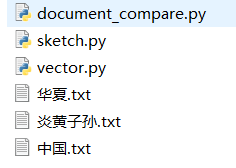document_compare.py
import sys
from vector import Vector
from sketch import Sketch

# 测试文档列表
filenames = ['华夏.txt', '中国.txt', '炎黄子孙.txt']
k = 5 #k-grams
d = 10000 #文档摘要维度
sketches = [0 for i in filenames]
for i in range(len(filenames)):
with open(filenames[i], 'r', encoding='UTF-8') as f:
sketches[i] = Sketch(text, k, d)

# 输出结果标题
print(' ' * 20, end = ' ')
for filename in filenames:
print('{:>25}'.format(filename), end = ' ')
print()

# 输出结果比较明细
for i in range(len(filenames)):
print('{:10}'.format(filenames[i]), end = ' ')
for j in range(len(filenames)):
print('{: <22}'.format(sketches[i].similarTo(sketches[j])), end = ' ')
print()

运行结果：
                       华夏.txt               中国.txt             炎黄子孙.txt
华夏.txt     0.9999999999999785     0.5011577874531165     0.42527486798147096
中国.txt     0.5011577874531165     1.0000000000000089     0.536571712819202
炎黄子孙.txt   0.42527486798147096    0.536571712819202      1.0000000000000757

结果表明，相同文档的相似度为1，相同类型的文档(华夏.txt和中国.txt)相似度尚可，而不同类型的文档(华夏.txt和炎黄子孙.txt)的相似度则稍微低些。
展开全文python 欧几里得距离
• c#文本相似度对比，亲测可用，可学习使用。主要用于对比出两个字符串中相似度能达到多少，项目中使用过已经经过优化
• 该文则首先建立文本集与码字集之间的 #.# 对应关系， 然后借用编码理论中汉明距离的概念， 由汉明距离的计算公式， 得到了一种全新的文本相似度的计算方法， 与传统的方法相比较， 它具有简便， 快速等优点。
• 词袋模型 from gensim import corpora from gensim import models ...# 1.1 历史比较文档的分词 all_location_list = [] for doc in location_list: doc_list = [word for word in jieba.cut_for_search(doc)]python 自然语言处理 nlp
• 在这篇博文中，作者比较了各种计算句子相似度的方法，并了解它们是如何操作的。词嵌入（word embeddings）已经在自然语言处理领域广泛使用，它可以让我们轻易地计算两个词语之间的语义相似性，或者找出与目标词语最...
• 1.1 eg.1: #-*- coding: utf-8 -*- #example 1： ...#最后计算corpus的向量相对于query的向量的余弦相似度，并排序输出。 #reference: https://blog.csdn.net/l7h9ja4/article/details/80220939...
• 因为在网页版比较相似度时，生成txt文档会耗费一定的时间，而且在Tomcat发布后路径不方便控制，所以取消txt文档的输入输出，全程以字符串形式输入和返回； 目前文档库中有100篇txt现代小说，若后期需要增加，直接...
• 向AI转型的程序员都关注了这个号????????????机器学习AI算法工程 公众号：datayxtext2vec, chinese text to vetor.(文本向量化表示工...
• gensim包的tfidf方法计算中文文本相似度，代码可直接运行，包含中文停用词，方便。
• java实现的文本相似度系统，使用向量空间模型以及余弦相似度距离公式，实测可以实现2篇文本的相似度计算且有一定的效果。
• 文本分析是数据挖掘的重要手段，利用文本分析，我们将很快的读取到一本书、一篇文章、一段话中的关键词和核心思想，而文本相似度就是我们用来剔除无用信息或者重复信息的重要手段，让计算机去找文本中的不同。...自然语言处理 算法
• 主要介绍了C#实现的字符串相似度对比类,本文直接给出类实现代码,代码中包含详细注释,需要的朋友可以参考下
• ## BERT文本相似度实战

千次阅读 热门讨论 2020-06-27 15:13:57
BERT文本相似度实战Bert 文本相似度实战（使用详解）参考资料代码准备数据建立config文件run_classifier.py 改造开始实践天天日日夜夜月月日夜近日改天提那天是否 Bert 文本相似度实战（使用详解） 大家好！其实 ...自然语言处理 tensorflow 机器学习 深度学习
• 这篇文章不是复制粘贴代码叫你怎么生成向量，怎么用余弦相似度计算文档的相似度（虽然可能也有这样的功能），而是尝试探究文档相似度比较的背后发生了什么。 也许从很多百度得到的资料，明白了两个向量是如何计算...gensim
• ## 文本相似度综述

千次阅读 2019-10-23 22:09:03
本文目录文本相似度的定义文本相似度计算方法基于字符串的方法基于语料库的方法基于词袋VSMLSA、PLSALDA（需要进一步了解）基于神经网络基于搜索引擎基于世界知识基于本体基于网络知识其他方法句法分析混合方法 文本...
• 使用 HanLP - 汉语言处理包 来处理，他能处理很多事情，如分词、调用分词器、命名实体识别、人名识别、地名识别、词性识别、篇章理解、关键词提取、简繁拼音转换、拼音转换、根据输入智能推荐、自定义分词器 ...java hanlp...